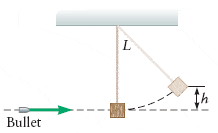# Impulse on a Pendulum with a Bullet

## Homework Statement

A bullet of mass 0.04kg is fired horizontally into a wooden block of mass 0.09kg. the wooden block is suspended from the ceiling by a long string. after an impact the bullet is embedded in the block and bullet and the block swing together until the block is 0.10m above its initial position.

a) Find the velocity of the bullet and block just after impact.

b) Find the initial velocity of the bullet## Homework Equations

these may not be the right equations but i am out of ideas

mu + MU = ( m + M ) v
( 1/ 2) (m + M ) v ^ 2 = ( m + M ) gh

## The Attempt at a Solution

This has taken question has taken me a long time and the method i used has given me a very unexpected answer...

I found some similar working online, but I'm not convinced.

mass of bullet m = 0.04 kg
mass of block M = 0.09 kg
maximum height reachs by block h = 0.01 m
from law of conservation of momentum , mu + MU = ( m + M ) v
mu + 0 = ( m + M ) v
where U = initial velocity of the block = 0
u = the initial speed of the bullet = ?
u = ( m+ M ) v / m
= 3.25 v ---( 1)
from law of conservation of energy ,
Kinetic energy of the bullet block system just after collision = potentia energy at maximumheight h
( 1/ 2) (m + M ) v ^ 2 = ( m + M ) gh
from this speed of the system just after collision v = √[ 2gh ]
= 1.4 m / s
plug this in eq ( 1) we get initial velocity of the bullet u = 3.25*1.4
= 4.55 m / s

is this right?

EDIT: i think the working goes wrong when i cancel out (m + M) from the equation ( 1/ 2) (m + M ) v ^ 2 = ( m + M ) gh

Last edited:

BUMP no one has any idea how to help me :( ?

can noone tell me where i went wrong? pretty please?

ideasrule
Homework Helper
( 1/ 2) (m + M ) v ^ 2 = ( m + M ) gh
from this speed of the system just after collision v = √[ 2gh ]
= 1.4 m / s
plug this in eq ( 1) we get initial velocity of the bullet u = 3.25*1.4
= 4.55 m / s

is this right?

That's right, if the wooden block really is only 0.09 kg. It makes sense: a real bullet would push a real 0.09 kg block way more than 0.1 cm.